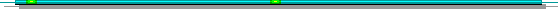Accueil Banque de problèmes récréatifs Récréations numériques Banque d'outils mathématiques Aide-mémoire Articles Références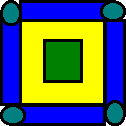Publications

 This is the third book published by Récréomath. Mathematical  Amusements Par Charles-É. Jean This edition includes 200 problems and their solution. The French version is published.

 Solutions 1 to 50 Problems 51 to 100 Solutions 51 to 100 Problems 101 to 150 Solutions 101 to 150 Problems 151 to 200 Solutions 151 to 200

***************
Problems 1 to 50
***************

 1

In the diagram, there are six squares and 17 matches.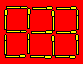Remove three matches and obtain four squares.

 2

James has 135 horses. He places his horses into five stalls. It is necessary that every stall to the right has one horse more than the stall to its left.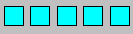Find the number of horses per stall.

 3

In this grid, write some numbers from 1 to 5. The numbers must be different in each line, column and diagonal.

 3 4 2 1 4 3 5 1

 4

On the board, each of the letters A, B, C, D and E corresponds to a different digit. The sum of each line is given on the right and the sum of each column at the bottom. When two letters are together, they form a two-digit number.

 E E E AB E E E AB E E E AB E E E AB AC AC AC CD

Find the value of E.

 5

In the circles, write two 2, three 3 and one 5. The result must be 4.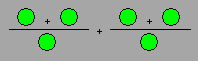6

In the addition, there are two missing numbers. The one is twice as great as the other.

 2 3 9 + 4 5 7 1 0 6 8

Find these two numbers.

 7

In a grid 5 ´ 5, Mary wants to write the numbers from 1 to 25. The sum must be the same one in each line and in each column.

 1 2 3 4 5 6 7 8 9 10 11 12 13 14 15 16 17 18 19 20 21 22 23 24 25

What is this sum ?

 8

Starting with cell 1 of the board, move the knight. It has to visit all the cells only once. The first five jumps are given.

 1 3 2 4 5

Number each cell from 6 to 25.

 9

Michael travels from G City to D City by crossing four different cities.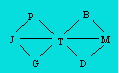Find a path that does not pass by B.

 10

In the addition, replace three digits by 1 so that the sum is 602.

 1 2 3 + 3 8 4 4 6 7 9 7 4

 11

In the circles, write numbers from 2 to 12. The product of the numbers of the circles joined by a straight line must be equal to 84.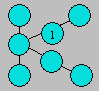12

Starting from K City, Martin wants to reach G City. In a city A, he delivers 25 automobiles, in B 22 automobiles, in C 18 automobiles, in D 10 automobiles. He stops exactly in four cities.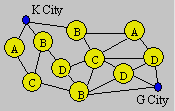Find the path that allows to deliver the most automobiles.

 13

In the grid, write six numbers from 10 to 100 in order to have different digits in each line, column and diagonal.

 87 69 23

 14

Four numbers and four operations are given.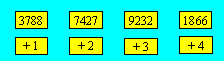Use each number and perform one of the four operations. You must obtain four numbers that are read horizontally and vertically.

 3 8

Write the new numbers in the grid.

 15

Peter put eight rabbits in each of the nine adjoining stalls. Later, the stall B is empty and the stall C contains 11 rabbits. However, there are always 24 rabbits in each horizontal, vertical and diagonal row.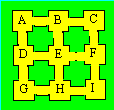Give the number of rabbits in the remaining stalls at that time.

 16

In the multiplication, find the missing digits.

 ? ? 9              2 ?         ? ? ? 1      1 ? ? ?      ?  ? 9 7 ?

 17

In the diagram, Lorena wants to write each of the numbers from 3 to 7. She placed the 8, the 9 and the 10.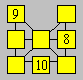Write the other numbers. The sum of the numbers of the cells joined by a straight line must be equal to 18.

 18

In this addition, George replaced each letter by a different digit. For example, S = 2, A = 4 and T = 3.

 M A T H        + E M A T         I Q U E S

What number corresponds to MATH ?

 19

299 + 399 is it divisible by 3 ?

 20

Each symbol represents a different digit. The sum of each line is given on the right and the sum of each column at the bottom. When two symbols are in the same square, they form a two-digit number.

 ( ( ( & ( ( ( ( & ( ( ( ( & ( & ( & ( & ( " (

Determine the value of (.

 21

In the addition, replace two digits by 2 so that the sum is 909.

 1 2 3 + 3 8 4 4 6 7 9 7 4

 22

In this figure, how many lozenges formed by four parts can you count ?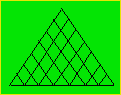23

In the circles, write each of the numbers from 1 to 6. The sum of the numbers that are joined by a straight line must be equal to 16.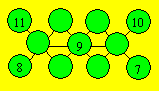24

You take the number 40. You make these operations in various orders : to add 5, to subtract 5, to multiply by 5 and to divide by 5.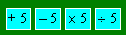Find the greatest result.

 25

In the circles, write the numbers 1, 2, 3, 4, 5 and 6. The result must be 2.26

Each letter corresponds to one of the digits given on the same line in the board. For example, F is equal to 2, 3 or 5.

 0 1 2 3 4 5 6 7 8 9 FUN * * * GAME * * * * SMILE * * * * * NAIL * * * * FINE * * * *

Find the word that corresponds to 780 976.

 27

Mark puts his 63 horses in nine stalls. The corner stalls contain 3, 6, 8 and 11 horses. The number of horses must be the same horizontally, vertically and diagonally.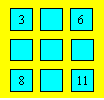Find the number of horses in each of the five empty stalls.

 28

Crystal chooses a two-digit number. She adds both digits : it is the value of A. She adds the number and its reversed. She divides the result by 11 : it is the value of B.

What is the value of B – A ?

 29

Using only once the digits below, find four 4-digit numbers that are divisible by 8.30

In the circles, write two 3, two 6 and two 7. The result must be 2.31

In the circles, write some numbers from 2 to 15. The product of the numbers of the circles joined by a straight line must be equal to 90.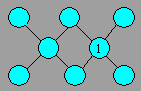32

In the addition, there are two missing numbers. One is the square of the other.

 2 5 8 + 2 5 8 1 0 6 8

Find these two numbers.

 33

In the series of six dominos, the upper row contains 20 points and the bottom row 12 points.

 ·      ·  · ·      ·       ·   ·      · ·      ·     ·      · ·      ·     ·      · ·      ·  · · ·    · ·       ·  · ·     · ·      ·  · ·    ·

Move two dominos in order to obtain 16 points per row.

 34

ABCD is a number of four different digits. We can write :

 A B     + C D        B A

What is the value of ABCD when C = 3 ?

 35

In the first board, each letter of the alphabet corresponds to a digit.

 1 a j s 4 d m v 7 g p y 2 b k t 5 e n w 8 h q z 3 c l u 6 f o x 9 i r

This second board contains a sentence.

 4 1 7 9 3 1 8 3 1 9 5 1 9 5 4 5 1 3 2 8 5 9 5 2 9 9 5 1 9 3 8 1 9 4 6 5 7 1 5 4 1 7 4 4 5 2 9 7 6 6 5 3 4 2 5 9 1

Decipher this sentence.

 36

In the grid, write some numbers from 1 to 7. The numbers must be different on each line and in each column.

 2 7 5 6 1 3 5 2 4 6 3 5 7 2 3 1 6

 37

Ask to a friend to choose a day and a month, to multiply the day by 12 and the number of the month by 31, to add the both products and to say the sum to you. For on July 6th, we write : (6 ´ 12) + (7 ´ 31) = 289.

Which is the month when the sum is 141 ?

 38

Write each of the numbers from 1 to 7, except 2, so that the sum is 14 in each row of three circles.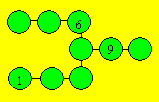39

In the squares, write each digit from 1 to 5. One of the numbers is a multiple of 5.

 + 5 1

Find three numbers where the sum is 51.

 40

This grid must contain five 5-digit numbers horizontally and the same numbers vertically.

 A B C D E A B C D 4 E

A : 19 106 ´ by 2, 4 or 6                     B : 9 979 ´ by 3, 5 or 7
C : 6 096 ´ by 6, 8 or 10                     D : 3 662 ´ by 5, 7 or 9
E : 3 373 ´ by 3, 13 or 33

Choosing the good products, fill in the grid.

 41

A farmer more fanciful than mathematician built a foot-bridge over a small river. The foot-bridge can support up to 72 kilos. The farmer weighs 71 kilos. He must cross the river with three balls of half a kilo each one.

How will he proceed ?

 42

How many numbers divisible by 2 can be formed with these four digits ?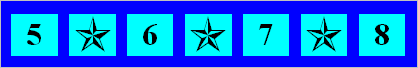43

In each of eight enclosures of a sheep-fold, there are six sheep.

 6 6 6 6 6 6 6 6

At a certain point, eight sheep escape. Then Steven rearranges the number of sheep per enclosure. There are still 18 sheep on each of four sides.

Show the new arrangment.

 44

The difference of the squares of two numbers is 224. Find these two numbers.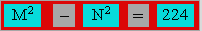45

In the circles, write some numbers from 2 to 15. The product of the numbers of the circles joined by a straight line must be equal to 120.46

Starting with the cell 1 of the board, move the knight until it visited all the cells only once. The first 10 jumps are given.

 1 9 7 10 2 8 6 3 5 4

Number each cell up to 36.

 47

Various objects of which the weight is expressed in whole numbers are placed on the perfectly balanced scales. The black circle weighs 10 grams.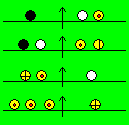Find the weight of each of the four other objects.

 48

In a store, there are 62 employees and seven departments. At a certain point, there are eight employees in a department and 26 employees in each of six rows of three departments.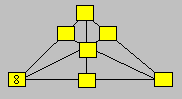Find the number of employees in each department.

 49

Each letter represents a different digit. When two letters are joined, they form a two-digit number.

 A + B = F     A ´ B = ED     D + B = B     A + A = ED